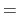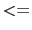Next: Materials Up: Coloring Methods Previous: Coloring by color categories   Contents   Index

## Color scale

Several of the coloring methods, including Beta', Charge', and Occupancy', describe a range of floating point values rather than a set of names. These are colored via the color scale, which is a list of 1024 smoothly changing colors. There are many color gradations available. All of them consist of transformations of three colors. For instance, RGB'' colors the smallest value red, values near the middle of the scale are green, and the largest values are blue. Colors in-between are linear mixes of the two colors. The list of available gradations is given below.

Table 6.4: Available Color Scale Gradations.
 Method Description RWB small=red, middle=white, large=blue BWR small=blue, middle=white, large=red RGryB small=red, middle=gray, large=blue BGryR small=blue, middle=gray, large=red RGB small=red, middle=green, large=blue BGR small=blue, middle=green, large=red RWG small=red, middle=white, large=green GWR small=green, middle=white, large=red GWB small=green, middle=white, large=blue BWG small=blue, middle=white, large=green BlkW small=black, large=white WBlk small=white, large=black

The minimum of the range of values is linearly scaled and shifted to start at 0 and end at 1. Assume the color scale is RGB. For a given value of x in the scale range [0..1], the RGB value is found first from a linear scaling based on the midpoint. If x0, R is 1 (for maximum red). This continues linearly until xmidpoint, at which point, R is 0 and stays 0. The green component is 0 at both x0 and x1 and is 1 at the midpoint. Linear scaling occurs in between. The blue component is 0 for xmidpoint, and 1 for x1.An additional term, `min'', is added to each of the component terms before they are merged. This shifts the final colors more towards white or black. Min can take on values from -1 to 1.There is only one color scale used at a time so it is impossible to display objects colored by multiple different color scales.Next: Materials Up: Coloring Methods Previous: Coloring by color categories   Contents   Index
vmd@ks.uiuc.edu